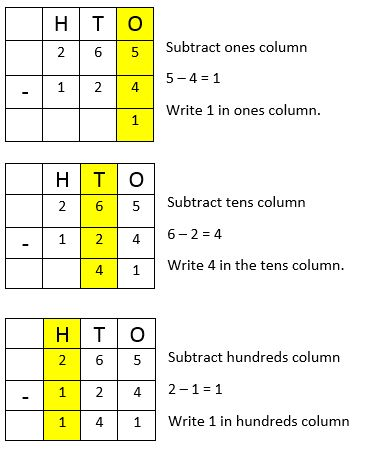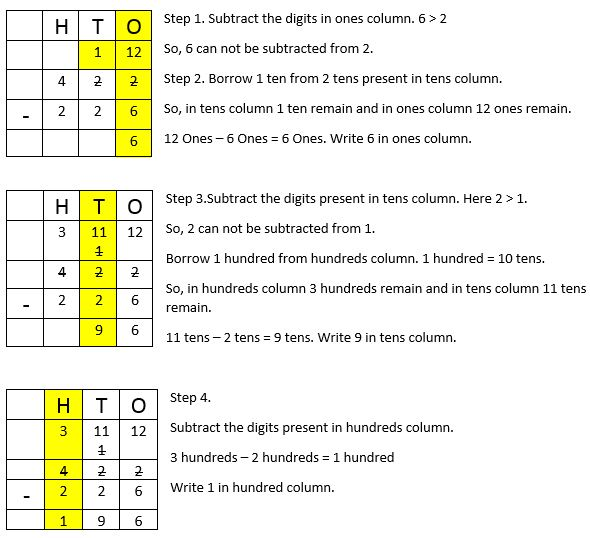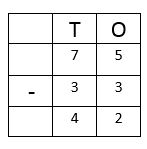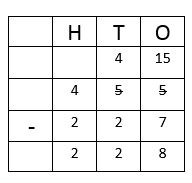## LetsPlayMaths.Com

WELCOME TO THE WORLD OF MATHEMATICS

# Class 2 Subtraction

Subtraction Without Borrowing

Subtraction With Borrowing

Story Time Subtraction

Subtraction Test

Subtraction Worksheet

## Subtraction Without Borrowing

This is the simplest form of subtraction, please follow the below mentioned instruction.

Step 1. Write both the numbers in tabular format. Larger number should remain at the top row.

Step 2. Subtract the digits present in once column and write the result in one’s column.

Step 3. Subtract the digits present in tens column and write the result in ten’s column.

Step 4. Subtract the digits present in hundred column and write the result in hundred’s column.

Let’s have a look at some examples.

Example 1. Subtract 35 from 87.

Solution. Write 87 and 35 in tabular format as shown below. Keep 87 in top row as it is greater than 35.So, the result is 52.

Example 2. Subtract 124 from 265.

Solution. Write 265 and 124 in tabular format as shown below. Keep 265 in the top row as it is greater than 124.So, the result is 141.

## Subtraction With Borrowing

Please follow the below mentioned instructions while performing this kind of subtraction.

Example 1. Subtract 25 from 50.

Solution. Write 50 and 25 in tabular format.Example 2. Subtract 226 from 422.

Solution. Write 422 and 226 in tabular format.So, the result is 196.

## Story Time Subtraction

In our day to day life, we use concept of subtraction in many ways, few examples are shown below.

Example 1.There were 75 chocolates in a packet. 33 chocolates distributed among children, then how many chocolates remained in the packet?Solution. Total number of chocolates = 75

Number of chocolates distributed = 33

Chocolates remained in the packet = Total number of chocolates – Chocolates distributes

= 75 – 33So, 42 chocolates remained in the packet.

Example 2. Total number of students in a school is 455. If the number of girls is 227, then what is the number of boys in the school?Solution. Total number of students = 455

Number of girls = 227

Number of boys = Total number of students – Total number of girls

= 455 - 227So, the number of boys is 228.

## Subtraction Test

Subtraction Test - 1

Subtraction Test - 2

Subtraction Test - 3

## Class-2 Subtraction Worksheet

Subtraction Worksheet - 1

Subtraction Worksheet - 2

Subtraction Worksheet - 3